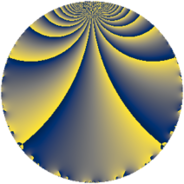# Properties

 Label 65.2.mLevel $65$ Weight $2$ Character orbit 65.m Rep. character $\chi_{65}(36,\cdot)$ Character field $\Q(\zeta_{6})$ Dimension $8$ Newform subspaces $1$ Sturm bound $14$ Trace bound $0$

# Related objects

## Defining parameters

 Level: $$N$$ $$=$$ $$65 = 5 \cdot 13$$ Weight: $$k$$ $$=$$ $$2$$ Character orbit: $$[\chi]$$ $$=$$ 65.m (of order $$6$$ and degree $$2$$) Character conductor: $$\operatorname{cond}(\chi)$$ $$=$$ $$13$$ Character field: $$\Q(\zeta_{6})$$ Newform subspaces: $$1$$ Sturm bound: $$14$$ Trace bound: $$0$$

## Dimensions

The following table gives the dimensions of various subspaces of $$M_{2}(65, [\chi])$$.

Total New Old
Modular forms 20 8 12
Cusp forms 12 8 4
Eisenstein series 8 0 8

## Trace form

 $$8q + 2q^{3} + 2q^{4} - 18q^{6} - 6q^{7} - 4q^{9} + O(q^{10})$$ $$8q + 2q^{3} + 2q^{4} - 18q^{6} - 6q^{7} - 4q^{9} - 2q^{10} + 20q^{12} + 4q^{14} - 6q^{15} - 2q^{16} - 2q^{17} + 12q^{19} + 12q^{20} - 12q^{22} - 10q^{23} - 12q^{24} - 8q^{25} + 10q^{26} - 4q^{27} - 18q^{28} - 8q^{29} + 4q^{30} + 6q^{32} + 42q^{33} + 10q^{35} + 20q^{36} + 6q^{37} - 16q^{38} - 12q^{40} + 12q^{41} + 4q^{42} - 2q^{43} - 42q^{46} + 28q^{48} + 12q^{49} - 8q^{51} - 6q^{52} - 24q^{53} + 18q^{54} + 12q^{56} + 36q^{58} - 12q^{59} - 28q^{61} + 4q^{62} - 24q^{63} - 8q^{64} - 8q^{65} + 12q^{66} + 6q^{67} - 14q^{68} - 16q^{69} - 48q^{72} + 10q^{74} - 2q^{75} + 54q^{76} - 36q^{77} - 56q^{78} - 16q^{79} + 8q^{81} + 4q^{82} - 30q^{84} + 18q^{85} + 22q^{87} - 18q^{88} + 24q^{89} + 40q^{90} + 28q^{91} + 44q^{92} + 32q^{94} - 16q^{95} - 30q^{97} + 72q^{98} + O(q^{100})$$

## Decomposition of $$S_{2}^{\mathrm{new}}(65, [\chi])$$ into newform subspaces

Label Dim. $$A$$ Field CM Traces $q$-expansion
$$a_2$$ $$a_3$$ $$a_5$$ $$a_7$$
65.2.m.a $$8$$ $$0.519$$ 8.0.22581504.2 None $$0$$ $$2$$ $$0$$ $$-6$$ $$q+(-1+\beta _{1}+\beta _{2}+\beta _{4}-\beta _{5})q^{2}+(\beta _{2}+\cdots)q^{3}+\cdots$$

## Decomposition of $$S_{2}^{\mathrm{old}}(65, [\chi])$$ into lower level spaces

$$S_{2}^{\mathrm{old}}(65, [\chi]) \cong$$ $$S_{2}^{\mathrm{new}}(13, [\chi])$$$$^{\oplus 2}$$